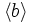# Baumslag-Solitar group:BS(1,2)

View a complete list of particular groups (this is a very huge list!)[SHOW MORE]

## Definition

This group is defined as the Baumslag-Solitar group with parameters 1 and 2, i.e., the group$BS(1,2)$. Explicitly, it is defined by means of the following presentation:$\langle a,b \mid bab^{-1} = a^2 \rangle$

## Group properties

Property Satisfied? Explanation
finitely generated group Yes See presentation above
finitely presented group Yes See presentation above
one-relator group Yes See presentation above
word-hyperbolic group No
solvable group Yes The normal closure of$a$ is isomorphic to the group$\mathbb{Z}[1/2]$, i.e., the group of 2-adic rationals. This is an abelian normal subgroup. The quotient group is isomorphic to$\langle b \rangle$, which is an abelian group isomorphic to the group of integers.
Noetherian group No The normal closure of$a$ is isomorphic to the subgroup$\mathbb{Z}[1/2]$, i.e., the group of 2-adic rationals. This is not finitely generated, because any finite generating set has an upper bound on possible denominators.
nilpotent group No
abelian group No
polycyclic group No
supersolvable group No